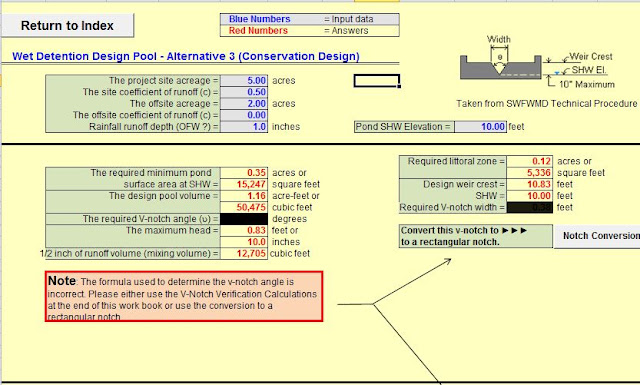# Drainage Calculations Excel Sheet - Free Spreadsheet## Drainage Calculation Index

14 Days Residence   :- This Sheet Calculates the Required Pond size at SHW for a 14days residence permanent wet pool.

Boring Calculations   :- This sheet calculates the required number of borings and hydraulic conductivity tests for a pond.

Buoyancy Calculations   :- This sheet verifies the required soil depth over a pond liner.

Channel Sections   :- This sheet calculates  geometric elements of various cross sections and segments of a circle.

Conversions-DRI and Metric   :- This sheet converts curve metric to English and vice versa and DRI to conductivity conversions.

Curve Number Calculations   :- This sheet converts curve number to coefficient of runoff numbers.

Effluent Filtration   :- This sheet verifies the recovery time for an effluent filtration system.

Intensity (Rainfall)   :- This sheet calculates rainfall intensity and allowable discharge in FDOT's zones 1 thorugh 11 for the 2 to 50 years events.

Kinematic Wave   :- This sheet calculates the time of concentration using the kinematic wave formula .

Littoral Shelf   :- This sheet calculates the required shelf size if the pond is larger than required.

Modified Rational   :- This sheet calculates the required storage volume for a 3 to 50 years storm even in all 11 FDOT zones .

NRCS Runoff Calculator :- This sheet calculates runoff by the NRCS method.

NRCS Tc Calculator   :-  This sheet calculates the NRCS time of concentration.

Orifice Bleeddown Calculator   :- This sheet calculates the required orifice size to discharge 1/2 thr treatment volume in 60 hours or more .

Pipe Calculations   :- This sheet calculates slopes, volumes based on surface area and elevation.

Pond Volume   :- This sheet calculates pond volumes based on surface area and elevation.

Rainfall Hydrograph Calculator   :-  This sheet generates rainfall hydro graphs for input into other programs.

Rating Curve Calculator    :- This sheet calculates rating curves for compound weirs for input into other programs.

Retention Basin Recovery    :- This sheet calculates retention basin recovery timeframes.

Sediment Sump Sizing   :- This sheet calculates the required sediment sump size.

Simplified Analytical Method   :-  This sheet provides a simplified pond (surface water management system) volume recovery analysis .

Tc Lag Method   :- This sheet calculates lag time.

V-Notch Calculation   :- This sheet verifies the 24 hours bleeddown requirment for a V-notch Weir.

Vegetative Upland Buffer   :- This sheet calculates vegetative upland buffers for a residential stromwater quality treatment alternative  practices.

Weir Calculations   :- This sheet calculates weir dimension based on height ,width or discharge rate,.

Weir Notch Flow    :- This sheet calculates the bleed down time for a weir notch.

## Disclaimer

These workbooks were created to assist in the review of common places calculations. These are not District approved or required. All users are the responsible for vaildating the accuracy of the internal calculations.If errors or omissions are noted within this workbook, please e-mail Richard M. Alt.P.E. at richard.alt@watermatters.org with specific information so that revisions can be made.